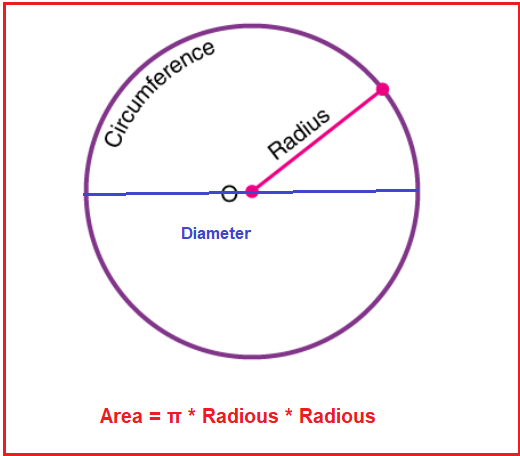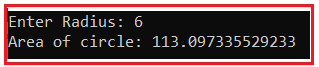# Area of Circle in C#

## Area of Circle Program in C# with Examples

In this article, I am going to discuss how to calculate the Area of a Circle in C# with Examples. Please read our previous article, where we discussed the Area of Triangle Program in C#. Here, in this article, first, we will discuss what is Circle, then we will discuss how to calculate the area of a circle and finally, we will see how to implement the area of circle program in C#.

##### What is a Circle?

A circle is a round-shaped figure that has no corners or edges. It has the set of all points on a plane that are a fixed distance from the center. Or, in geometry, a circle can be defined as a closed, two-dimensional curved shape.

##### How to calculate the area of a Circle?

Following is the formula to calculate the area of the Circle.

Area of a circle( A) = π * r * r
where r is the radius of the circle.
A is the area of the Circle
π is the constant value I.e. (22/7 or 3.14)
r=d/2; i.e. If d=4 given then radius=2Example:
Input: r=7
Area of a circle= π * r * r
= (22/7) *7*7
=154

In this program, we are going to use the following data type and constants.

1. Double: Double is also a data type that is used to represent the floating-point numbers. It has 15 decimal digits of precision. Example: double y = 4244.546
2. Float: Floating-point numbers are used for decimal and exponential values. It is a primitive data type. It has 6 decimal digits of precision. Example: float n2 = 5.5
3. PI: pi is a constant variable that value is 3.14. It is used for mathematical calculation. Example: Pi=3.14(decimal form) and Pi=22/7(fraction form)
##### Logic to Calculate the Area of Circle in C#

To find the area of Circle, we need to follow the below steps.

1. First, we will take variable radius(r).
2. Then we will constant variable pi.
3. After that, we will calculate the area of the circle by using the formula: π * r * r
4. And the result will be store in the area variable.
##### Example: Area of Circle Program in C#.

The following C# Program will calculate the area of the Circle.

```using System;
class Circle
{
static void Main(string[] args)
{